# The SIMILARITY Procedure

### TARGET Statement

• TARGET variable-list < / options> ;

The TARGET statement lists the numeric target variables in the DATA= data set whose values are to be accumulated to form the time series or represent ordered numeric sequences (when no ID statement is specified).

An input data set variable can be specified in only one INPUT or TARGET statement. Any number of TARGET statements can be used. The following options can be used with a TARGET statement:

ACCUMULATE=option

specifies how the data set observations are accumulated within each time period for the variables listed in the TARGET statement. If the ACCUMULATE= option is not specified in the TARGET statement, accumulation is determined by the ACCUMULATE= option in the ID statement. If the ACCUMULATE= option is not specified in the ID statement or the TARGET statement, no accumulation is performed. See the ID statement ACCUMULATE= option for more details.

COMPRESS=option | (options)

specifies the sliding sequence (global) and warping (local) compression range of the target sequence with respect to the input sequence. Compression of the target sequence is the same as expansion of the input sequence and vice versa. The compression limits are defined based on the length of the target sequence and are imposed on the target sequence. The following compression options are provided:

GLOBALABS=integer

specifies the absolute global compression, where integer ranges from zero to 10,000. GLOBALABS=0 implies no global compression, which is the default unless the GLOBALPCT= option is specified.

GLOBALPCT=number

specifies global compression as a percentage of the length of the target sequence, where number ranges from zero to 100. GLOBALPCT=0 implies no global compression, which is the default. GLOBALPCT=100 implies maximum allowable compression.

LOCALABS=integer

specifies the absolute local compression, where integer ranges from zero to 10,000. The default is maximum allowable absolute local compression unless the LOCALPCT= option is specified.

LOCALPCT=number

specifies local compression as a percentage of the length of the target sequence, where number ranges from zero to 100. The percentage specified by the LOCALPCT= option must be less than the GLOBALPCT= option. LOCALPCT=0 implies no local compression. LOCALPCT=100 implies maximum allowable local compression. The default is LOCALPCT=100.

If the SLIDE=NONE or the SLIDE=SEASON option is specified in the TARGET statement, the global compression options are ignored. To disallow local compression, use the option COMPRESS=(LOCALPCT=0 LOCALABS=0).

If the SLIDE=INDEX option is specified, the global compression options are not ignored. To completely disallow both global and local compression, use the option COMPRESS=(GLOBALPCT=0 LOCALPCT=0) or COMPRESS=(GLOBALABS=0 LOCALABS=0). To allow only local compression, use the option COMPRESS=(GLOBALPCT=0 GLOBALABS=0). These are the default compression options.

The preceding options can be used in combination to specify the desired amount of global and local compression as the following examples illustrate, where
denotes the global compression limit and
denotes the local compression limit:

• COMPRESS=(GLOBALPCT=20) allows the global and local compression to range from zero to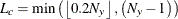.

• COMPRESS=(GLOBALPCT=20 GLOBALABS=10) allows the global and local compression to range from zero to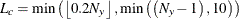.

• COMPRESS=(LOCALPCT=10) allows the local compression to range from zero to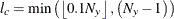.

• COMPRESS=(LOCALPCT=20 LOCALABS=5) allows the local compression to range from zero to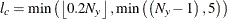.

• COMPRESS=(GLOBALPCT=20 LOCALPCT=20) allows the global compression to range from zero toand allows the local compression to range from zero to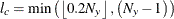.

• COMPRESS=(GLOBALPCT=20 GLOBALABS=10 LOCALPCT=10 LOCALABS=5) allows the global compression to range from zero toand allows the local compression to range from zero to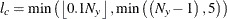.

Suppose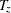is the length of the input time series and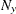is the length of the target sequence. The valid global compression limit,, is always limited by the length of the target sequence: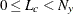.

Suppose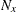is the length of the input sequence andis the length of the target sequence. The valid local compression limit,, is always limited by the lengths of the input and target sequence: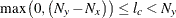.

DIF=(numlist)

specifies the differencing to be applied to the accumulated time series. The list of differencing orders must be separated by spaces or commas. For example, DIF=(1,3) specifies first, then third, order differencing. Differencing is applied after time series transformation. The TRANSFORM= option is applied before the DIF= option. Simple differencing is useful when you want to detrend the time series before computing the similarity measures.

EXPAND=option | (options)

specifies the sliding sequence (global) and warping (local) expansion range of the target sequence with respect to the input sequence. Expansion of the target sequence is the same as compression of the input sequence and vice versa. The expansion limits are defined based on the length of the input sequence, but are imposed on the target sequence. The following expansion options are provided:

GLOBALABS=integer

specifies the absolute global expansion, where integer ranges from zero to 10,000. GLOBALABS=0 implies no global expansion, which is the default unless the GLOBALPCT= option is specified.

GLOBALPCT=number

specifies global expansion as a percentage of the length of the target sequence, where number ranges from zero to 100. GLOBALPCT=0 implies no global expansion, which is the default unless the GLOBALABS= option is specified. GLOBALPCT=100 implies maximum allowable global expansion.

LOCALABS=integer

specifies the absolute local expansion, where integer ranges from zero to 10,000. The default is the maximum allowable absolute local expansion unless the LOCALPCT= option is specified.

LOCALPCT=number

specifies local expansion as a percentage of the length of the target sequence, where number ranges from zero to 100. LOCALPCT=0 implies no local expansion. LOCALPCT=100 implies maximum allowable local expansion. The default is LOCALPCT=100.

If the SLIDE=NONE or the SLIDE=SEASON option is specified in the TARGET statement, the global expansion options are ignored. To disallow local expansion, use the option EXPAND=(LOCALPCT=0 LOCALABS=0).

If the SLIDE=INDEX option is specified, the global expansion options are not ignored. To completely disallow both global and local expansion, use the option EXPAND=(GLOBALPCT=0 LOCALPCT=0) or EXPAND=(GLOBALABS=0 LOCALABS=0). To allow only local expansion, use the option EXPAND=(GLOBALPCT=0 GLOBALABS=0). These are the default expansion options.

The preceding options can be used in combination to specify the desired amount of global and local expansion as the following examples illustrate, where
denotes the global expansion limit and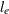denotes the local expansion limit:

• EXPAND=(GLOBALPCT=20) allows the global and local expansion to range from zero to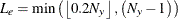.

• EXPAND=(GLOBALPCT=20 GLOBALABS=10) allows the global and local expansion to range from zero to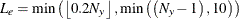.

• EXPAND=(LOCALPCT=10) allows the local expansion to range from zero to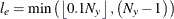.

• EXPAND=(LOCALPCT=10 LOCALABS=5) allows the local expansion to range from zero to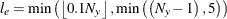.

• EXPAND=(GLOBALPCT=20 LOCALPCT=10) allows the global expansion to range from zero toand allows the local expansion to range from zero to.

• EXPAND=(GLOBALPCT=20 GLOBALABS=10 LOCALPCT=10 LOCALABS=5) allows the global expansion to range from zero toand allows the local expansion to range from zero to.

Supposeis the length of the input time series andis the length of the target sequence. The valid global expansion limit,, is always limited by the length of the input time series: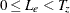.

Supposeis the length of the input sequence andis the length of the target sequence. The valid local expansion limit,, is always limited by the lengths of the input and target sequence: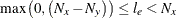.

MEASURE=option

specifies the similarity measure to be computed by using the working input and target sequences. The following similarity measures are provided:

SQRDEV

squared deviation. This option is the default.

ABSDEV

absolute deviation

MSQRDEV

mean squared deviation

MSQRDEVINP

mean squared deviation relative to the length of the input sequence

MSQRDEVTAR

mean squared deviation relative to the length of the target sequence

MSQRDEVMIN

mean squared deviation relative to the minimum valid path length

MSQRDEVMAX

mean squared deviation relative to the maximum valid path length

MABSDEV

mean absolute deviation

MABSDEVINP

mean absolute deviation relative to the length of the input sequence

MABSDEVTAR

mean absolute deviation relative to the length of the target sequence

MABSDEVMIN

mean absolute deviation relative to the minimum valid path length

MABSDEVMAX

mean absolute deviation relative to the maximum valid path length

User-Defined

The measure is computed by a user-defined function created by using the FCMP procedure, where User-Defined is the function name.

NORMALIZE=option

specifies the sequence normalization to be applied to the working target sequence. The following normalization options are provided:

NONE

No normalization is applied. This option is the default.

ABSOLUTE

Absolute normalization is applied.

STANDARD

Standard normalization is applied.

User-Defined

Normalization is computed by a user-defined subroutine that is created by using the FCMP procedure, where User-Defined is the subroutine name.

PATH=option

specifies the similarity measure and warping path information to be computed using the working input and target sequences. The following similarity measures and warping path are provided:

User-Defined

The measure and path are computed by a user-defined subroutine that is created by using the FCMP procedure, where User-Defined is the subroutine name

For computational efficiency, the PATH= option should be only used when you want to compute both the similarity measure and the warping path information. If only the similarity measure is needed, use the MEASURE= option. If you specify both the MEASURE= and PATH= option in the TARGET statement, the PATH= option takes precedence.

SDIF=(numlist)

specifies the seasonal differencing to be applied to the accumulated time series. The list of seasonal differencing orders must be separated by spaces or commas. For example, SDIF=(1,3) specifies first, then third, order seasonal differencing. Differencing is applied after time series transformation. The TRANSFORM= option is applied before the SDIF= option. Seasonal differencing is useful when you want to deseasonalize the time series before computing the similarity measures.

SETMISSING=option | number
SETMISS=option | number

option specifies how missing values (either actual or accumulated) are interpreted in the accumulated time series for variables that are listed in the TARGET statement. If the SETMISSING= option is not specified in the TARGET statement, missing values are set based on the SETMISSING= option in the ID statement. If the SETMISSING= option is not specified in the ID statement or the TARGET statement, no missing value interpretation is performed. See the ID statement SETMISSING= option for more details.

SLIDE=option

specifies the sliding of the target sequence with respect to the input sequence. The following slides are provided:

NONE

No sequence sliding. The input time series is compared with the target sequence directly with no sliding. This option is the default.

INDEX

Slide by time index. The input time series is compared with the target sequence by observation index.

SEASON

Slide by seasonal index. The input time series is compared with the target sequence by seasonal index.

The SLIDE= option takes precedence over the COMPRESS= and EXPAND= options.

TRANSFORM=option

specifies the time series transformation to be applied to the accumulated time series. The following transformations are provided:

NONE

No transformation is applied. This option is the default.

LOG

Logarithmic transformation is applied.

SQRT

Square-root transformation is applied.

LOGISTIC

Logistic transformation is applied.

BOXCOX(number)

Box-Cox transformation with parameter is applied, where the real number is between –5 and 5

User-Defined

Transformation is computed by a user-defined subroutine that is created by using the FCMP procedure, where User-Defined is the subroutine name.

When the TRANSFORM= option is specified, the time series must be strictly positive unless a user-defined function is used.

TRIMMISSING=option
TRIMMISS= option

specifies how missing values (either actual or accumulated) are trimmed from the accumulated time series or ordered sequence for variables that are listed in the TARGET statement. The following trimming options are provided:

NONE

No missing value trimming is applied.

LEFT

Beginning missing values are trimmed.

RIGHT

Ending missing values are trimmed.

BOTH

Both beginning and ending missing values are trimmed. This is the default.

ZEROMISS=option

specifies how beginning and ending zero values (either actual or accumulated) are interpreted in the accumulated time series or ordered sequence for variables listed in the TARGET statement. If the ZEROMISS= option is not specified in the TARGET statement, beginning and ending values are set based on the ZEROMISS= option in the ID statement. See the ID statement ZEROMISS= option for more details.